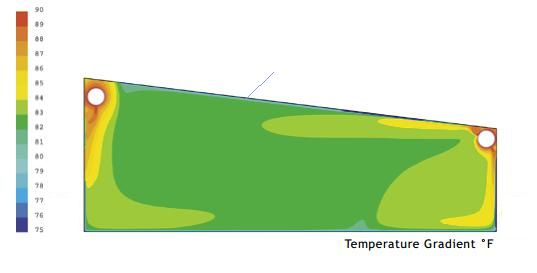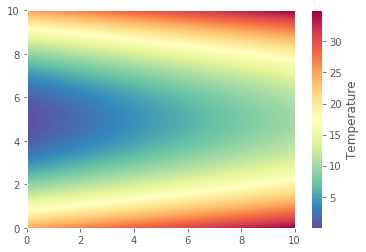#### 怎么用Python画这种温度分布图

统计/机器学习 数据可视化 Python    浏览次数：8144        分享

1GodStick   2018-09-14 08:50

##### 1个回答
4

import matplotlib.pyplot as plt
import numpy as np
# 生成数据
X, Y = np.meshgrid(np.linspace(0, 10, 1000), np.linspace(0, 10, 1000))
temperature = X + (Y - 5) ** 2

# 作图
fig = plt.figure()
c = ax.pcolormesh(X, Y, temperature, cmap='Spectral_r')
cb = fig.colorbar(c)
cb.set_label('Temperature')
plt.show()SofaSofa数据科学社区DS面试题库 DS面经wxw_pku   2018-09-14 09:58相关主题

python画散点图   1回答